Courses

# Test: Sequencing And Scheduling

## 28 Questions MCQ Test Manufacturing and Industrial Engineering | Test: Sequencing And Scheduling

Description
This mock test of Test: Sequencing And Scheduling for Mechanical Engineering helps you for every Mechanical Engineering entrance exam. This contains 28 Multiple Choice Questions for Mechanical Engineering Test: Sequencing And Scheduling (mcq) to study with solutions a complete question bank. The solved questions answers in this Test: Sequencing And Scheduling quiz give you a good mix of easy questions and tough questions. Mechanical Engineering students definitely take this Test: Sequencing And Scheduling exercise for a better result in the exam. You can find other Test: Sequencing And Scheduling extra questions, long questions & short questions for Mechanical Engineering on EduRev as well by searching above.
QUESTION: 1

Solution:
QUESTION: 2

Solution:
QUESTION: 3

### Tardiness is the measure of

Solution:
QUESTION: 4

Which of the following is not an assumption of scheduling and sequencing?

Solution:
QUESTION: 5

The number of possible ways for ‘n’ jobs to sequence

Solution:
QUESTION: 6

If critical ratio is < 1 then

Solution:
QUESTION: 7

Which of the following minimizes the idle time of an n-jobs and 2 machine problem?

Solution:
QUESTION: 8

Least slack is given by

Solution:
QUESTION: 9

Critical ratio is given by

Solution:
QUESTION: 10

Flow time is given by

Solution:
QUESTION: 11

Apply FCFS rule and find the mean flow time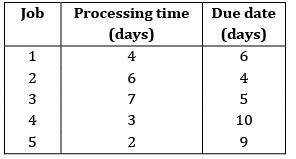Solution:

Sequence for FCFS will be 1 − 2 − 3 − 4 − 5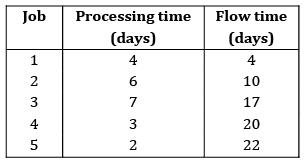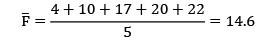QUESTION: 12

The following jobs are to be processed on a machine as per the data listed in the table below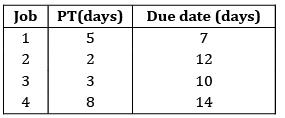Using SPT rule, the total tardiness is _____________

Solution:

Sequence using SPT: 2 − 3 − 1 − 4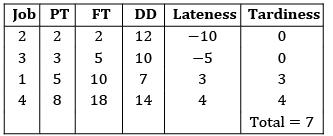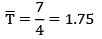QUESTION: 13

The sequence that would minimize the make span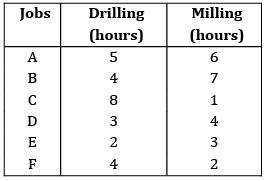Solution:

Flow time will be minimum with SPT sequence = D − B − A − C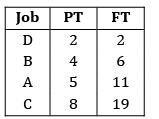Total FT = 38

Minimum cost = 38 × 50 = Rs. 1900

*Answer can only contain numeric values
QUESTION: 14

Seven jobs arrived in a sequence as given belowCalculate average flow time (in days) for the above jobs using SPT rule.

Solution: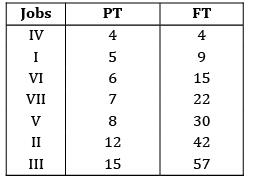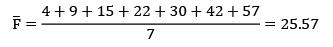QUESTION: 15

Four jobs has to be processed on a single machine. The details are given in following table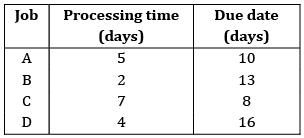The average lateness using SPT rule is ___________

Solution: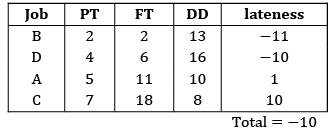Average = −3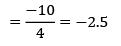QUESTION: 16

A defense contractor has six different jobs in process with the delivery requirements shown. Today is day (60) and if the contractor uses a critical ratio method for sequencing, find the sequence.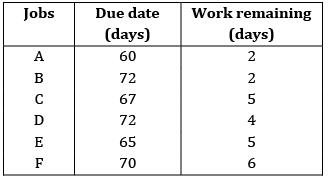Ans. C

Solution: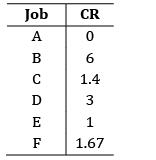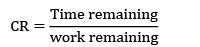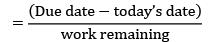Job sequence = A − E − C − F − D − B

QUESTION: 17

Four jobs have to be sequenced on a single facility with the objective of minimizing the maximum lateness.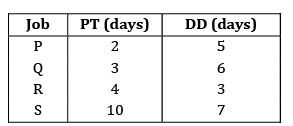The last job that should be taken up

Solution:

EDD rule minimizes the max-lateness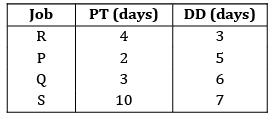QUESTION: 18

Consider the following data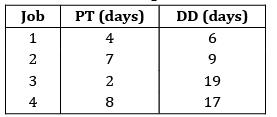The no. of tardy jobs using SPT rule

Solution:

Sequence using SPT: 3 − 1 − 2 − 4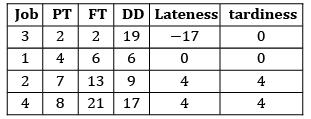No. of tardy jobs = 2

QUESTION: 19

Consider the following data for a job-shop problem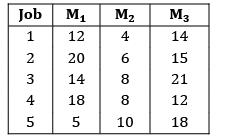The optimal sequence for the above problem is

Solution:

Convert the given problem into a two machine problem and apply Johnson’s algorithm

Max {M2} ≤ min{M1} or min{M3}

10 ≤ 5 ✘

10 ≤ 12✓

The problem can be converted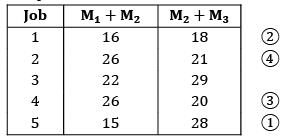Applying Johnson’s algorithm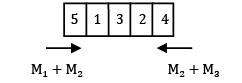QUESTION: 20

Using Johnson’s rule find the optimal sequence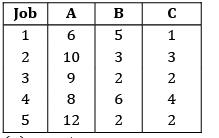Solution:

Convert 3 machine problem into a two machine problem and apply Johnson’s rule

Max {M2} ≤ min{M1} or min{M3}

6 ≤ 6✓

6 ≤ 1✘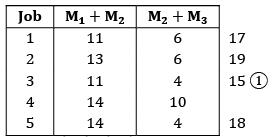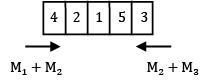QUESTION: 21

Four jobs has to be processed on a single machine. The details are given in the following data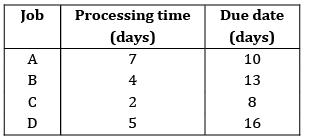The minimum average tardiness is.

Solution:

The average tardiness is minimized when EDD rule is used sequence using EDD rule C −A − B − D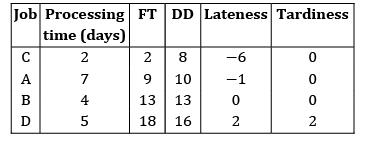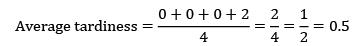QUESTION: 22

Four jobs need to be processed sequentially on two machines, first on Machine X and then on Machine Y. Each machine can process only one job at a time. The processing times in(hours) are given below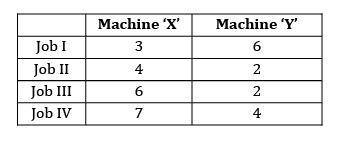The make span of the jobs is ____________

Solution:

Apply Johnson’s algorithm to find the make span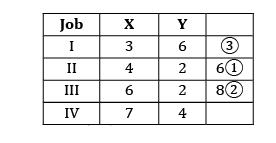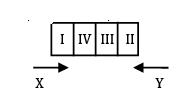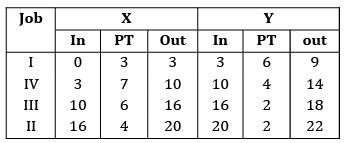*Answer can only contain numeric values
QUESTION: 23

Consider the following data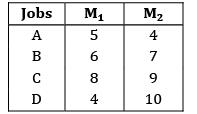What will be the earliest time that B can be delivered to the customer?

Solution:

According to Johnson’s rule, the optimal sequence is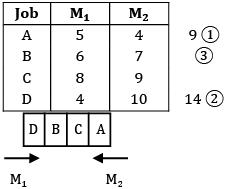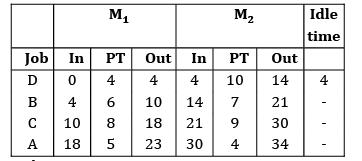Make span = 34

Job B can be delivered at the end of 21 hours

*Answer can only contain numeric values
QUESTION: 24

Consider the following data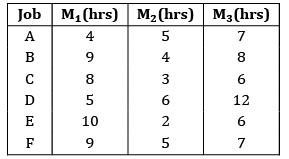The optimal sequence using Johnson’s rule is A − D − B − F − C − E. Find the make span.

Solution: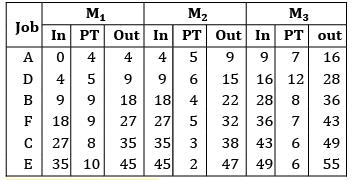Make span = 55 hours

QUESTION: 25

The todays date in a production calendar of an industry is 175. Sequence the jobs listed below by critical ratio rule and also find the number of jobs that are behind the schedule.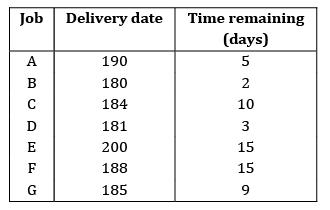Solution: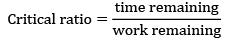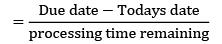Jobs should be arranged in the increasing order of critical ratio.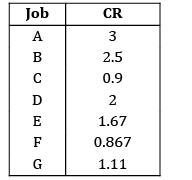Sequence: F − C − G − E − D − B − A

Number of jobs behind schedule = no. of jobs whose critical ratio is less than 1

i.e., C & F in this case

∴ Number of jobs = 2

*Answer can only contain numeric values
QUESTION: 26

Consider the following 2 job and M-Machine problem

Job1 Lathe (2 hours) → Milling (1 hour)→→Drilling (4hours)

Job 2 Milling (1 hour)→ Lathe (3 hours) → Drilling (3 hours)

Find the make span.

Solution: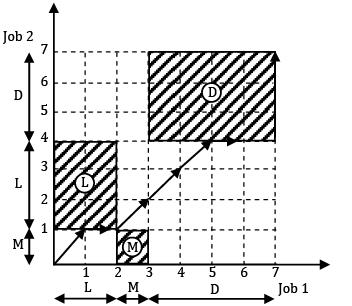Make span = 10 hours

*Answer can only contain numeric values
QUESTION: 27

Using graphical method, determine the minimum time needed to process the two jobs on four machines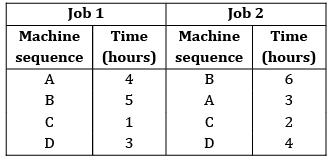Solution: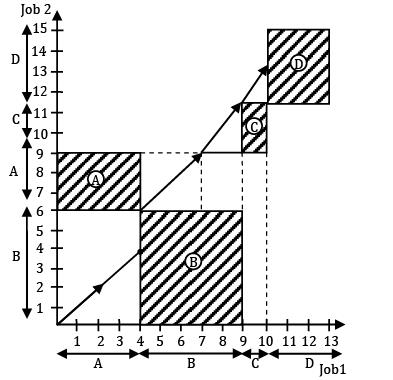Total completion time = 4+2+5+7+3 = 15

= 15 hours

QUESTION: 28

There are six cars waiting to be repaired at a car repair shop. Each car has first to be dented and then painted. The estimated times for each car are given below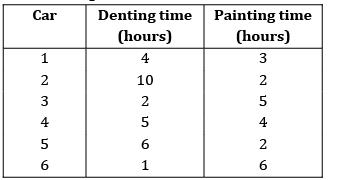Find the total idle time for machine.

Solution: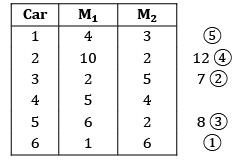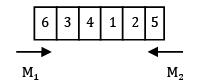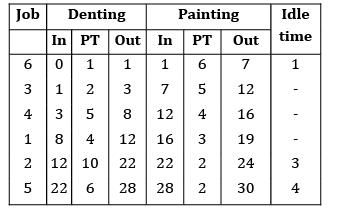Idle time = 8 hours